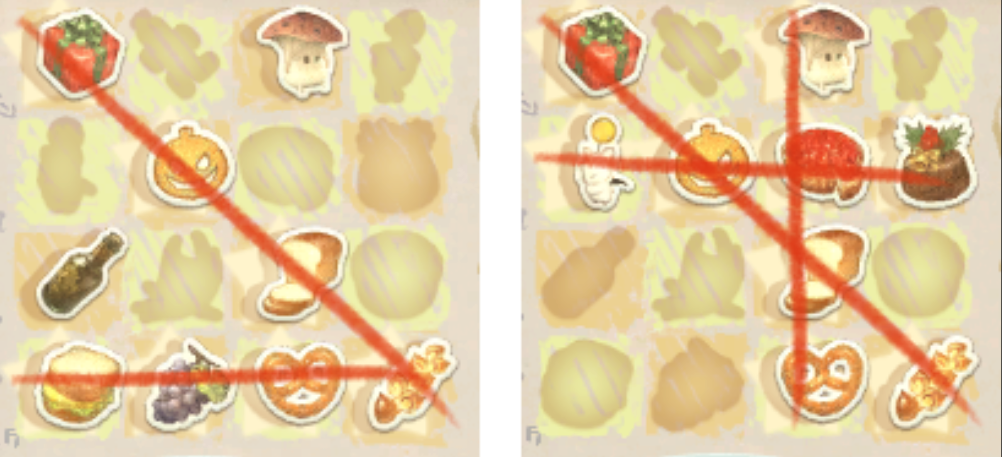# 2021 TPC 腾讯程序设计竞赛 热身赛

## 零、背景

2021 年 TPC 腾讯程序设计竞赛 又开始了。

## 一、Future of Tencent

``````int main() {
int t;
scanf("%d", &t);
while (t--) {
int n;
scanf("%d", &n);
if (n) {
printf("You are the future of Tencent!\n");
} else {
printf("Good luck and Enjoy TPC!\n");
}
}
return 0;
}
``````

## 二、Wondrous Tails``````int main() {
init();
// printf("num=%d\n", num);

int t;
scanf("%d", &t);
while (t--) {
char str;
for (int i = 0; i < 4; i++) {
scanf("%s", str[i]);
}

int ans = 9;
for (int k = 0; k < num; k++) {
int tmpAns = 9;
for (int i = 0; i < 4; i++) {
for (int j = 0; j < 4; j++) {
if (base[k][i][j] == str[i][j]) {
tmpAns--;
}
}
}
ans = min(ans, tmpAns);
}

printf("%d\n", ans);
}

return 0;
}
``````

## 三、BFS Sequence

``````
vector<int> one(100011, 0);
vector<int> two(100011, 0);
vector<int> pre(100011, 0);

int main() {
int t;
scanf("%d", &t);
while (t--) {
int n;
scanf("%d", &n);
for (int i = 0; i < n; i++) {
scanf("%d", &one[i]);
}
for (int i = 0; i < n; i++) {
scanf("%d", &two[i]);
}

if (one != two) {
printf("No\n");
continue;
}

int root = 0;

int onePos = 0;
int twoPos = 0;
unordered_set<int> oneSet;
unordered_set<int> twoSet;
for (int i = 0; i < n;) {
onePos = twoPos = i;
int tmpRoot = one[onePos];
oneSet.clear();
twoSet.clear();

do {
// printf("%d %d\n", onePos, twoPos);
int tmpVal = one[onePos];
pre[tmpVal] = root;

oneSet.insert(tmpVal);
if (twoSet.count(tmpVal)) {
onePos++;
continue;
}

while (twoPos < n && two[twoPos] != tmpVal) {
twoSet.insert(two[twoPos]);
twoPos++;
}
if (twoPos < n && two[twoPos] == tmpVal) {
twoSet.insert(two[twoPos]);
twoPos++;
}
} while (oneSet.size() != twoSet.size());
i = twoPos;
root = tmpRoot;
// printf("root=%d\n", root);
}

printf("Yes\n");
for (int i = 1; i <= n; i++) {
printf("%d%c", pre[i], i < n ? ' ' : '\n');
}
}

return 0;
}
``````

## 四、Persistent String

1）`+ p t`，在位置 p 之后插入字符串 t。
2）`! a b c p`，将字符串子串 `s[a,b]` 在位置 p 之后重复插入 c 次。
3）`? p` 询问位置 p 的字符。

``````map<int, pair<LL, char>> ans;

int main() {
int t, q;
scanf("%d", &t);
while (t--) {
input(q);
ans.clear();
for (int i = q; i >= 0; i--) {
Op* p = ops + i;
LL pos = p->pos;

if (p->op == '?') {
ans[i].first = pos - 1;
continue;
}

LL len = 0;
if (p->op == '+') {
len = strlen(p->pt);
} else {
len = (p->b - p->a + 1) * p->c;
}

for (auto& kv : ans) {
auto& pp = kv.second;
if (pp.first == -1 || pp.first < pos) {
// do nothing
} else if (pos <= pp.first && pp.first < pos + len) {
if (p->op == '+') {
pp.second = p->pt[pp.first - pos];
pp.first = -1;
} else {
pp.first = p->a - 1 + (pp.first - pos) % (p->b - p->a + 1);
}
} else {
pp.first -= len;
}
}
}
for (auto& kv : ans) {
printf("%c\n", kv.second.second);
}
}

return 0;
}
``````

《完》

-EOF-

## 关注公众号,接收最新消息

1.